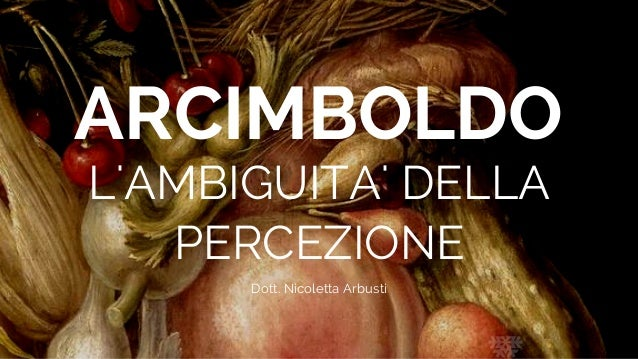Successfully reported this slideshow.Upcoming SlideShare
×

# Arcimboldo – l’ambiguità della percezione

261 views

Published on

Slide della conferenza
” Arcimboldo – l’ambiguità della percezione “ tenuta da Nicoletta Arbusti in data 10 novembre 2017
presso Palazzo Medici Riccardi

“La Trilogia delle Insalate “
prima conferenza

Published in: Art & Photos
• Full Name
Comment goes here.

Are you sure you want to Yes NoAre you sure you want to  Yes  No
• Be the first to like this

### Arcimboldo – l’ambiguità della percezione

1. 1. ARCIMBOLDO L'AMBIGUITA' DELLA PERCEZIONE Dott. Nicoletta Arbusti
2. 2. ROLAND BARTHES " L ' o v v i o e l ' o t t u s o - A r c i m b o l d o " E d . A b s c o n d i t a 2 0 0 5
3. 3. GIUSEPPE ARCIMBOLDO O ARCIMBOLDI A u t o r i t r a t t o c a . 1 5 7 5 - N a r o d n i G a l e r i e , P r a g u e
4. 4. BENNO GEIGER " I d i p i n t i g h i r i b i z z o s i d i G i u s e p p e A r c i m b o l d i " E d . V a l l e c c h i 1 9 5 4
5. 5. I q u a t t r o e l e m e n t i   d i   A r c i m b o l d o ACQUA ARIA FUOCO1566 COLLEZIONE PRIVATA 1568 KUNSTHISTORISCHES MUSEUM, VIENNA TERRA1566 COLLEZIONE PRIVATA 1570 VIENNA, LICHTENSTEIN - THE PRINCELY COLLECTIONS
6. 6. ROLAND BARTHES " L ' o v v i o e l ' o t t u s o - A r c i m b o l d o " E d . A b s c o n d i t a 2 0 0 5
7. 7. VERTUMNO r i t r a t t o d e l l ' I m p e r a t o r e 1 5 9 0 - C a s t e l l o d i S k o k l o s t e r S v e z i a
8. 8. L e q u a t t r o s t a g i o n i   d i   A r c i m b o l d o INVERNO PRIMAVERA ESTATE 1572 DENVER, DENVER ART MUSEUM 1572 HOUSTON, THE MENIL COLLECTION AUTUNNO 1555-1560 CA MONACO DI BAVIERA, BAYERISCHE STAATSGEMÄLDESAMMLUNGEN 1572 DENVER, DENVER ART MUSEUM
9. 9. L'ORTOLANO (PRIAPO)  CIOTOLA DI VERDURE (1590-1593 CIRCA) CREMONA, MUSEO CIVICO “ALA PONZONE” IL CUOCO / PIATTO DI ARROSTO STOCCOLMA, NATIONALMUSEUM L e F i g u r e R e v e r s i b i l i   d i   A r c i m b o l d o
10. 10. INVERNO 1 5 7 2 H o u s t o n , T h e M e n i l C o l l e c t i o n
11. 11. SEMIR ZECHI N e u r o b i o l o g o I s t i t u t o d i N e u r o e s t e t i c a U n i v e r s i t à d i B e r k e l e y C a l i f o r n i a " L a v i s i o n e d a l l ' i n t e r n o " E d . B o l l a t i B o r i n g h i e r i 2 0 1 0
12. 12. EVA ADAMO 1 5 7 8 C o l l e z i o n e p r i v a t a
13. 13. ARTHUR SCHOPENHAUER F i l o s o f o " I l m o n d o c o m e v o l o n t à e r a p p r e s e n t a z i o n e " N e w t o n C o m p t o n E d i t o r i 2 0 1 5
14. 14. FA PAURA MA È VERAMENTE UNA BUONA PERSONA 1 8 4 7 U t a g a w a K u n i y o s h i (MIKAKE WA KOWAI GA TONDA II HITO DA) THE CAT DEMON ON THE TOKAIDO ROAD 1 8 5 0 c a (TOKAIDO NEKO NO SEI)
15. 15. VILANAYUR RAMACHANDRAN N e u r o s c i e n z i a t o d e l c o m p o r t a m e n t o e d e l l a p s i c o f i s i c a , i n p a r t i c o l a r e d e l l a v i s i o n e . " C h e c o s a s a p p i a m o d e l l a m e n t e " M o n d a d o r i E d i t o r i 2 0 0 4
16. 16. GRAZIEDott. Nicoletta Arbusti